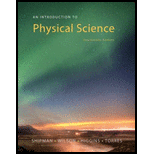Chapter 1, Problem 21EAn Introduction to Physical Science

14th Edition
James Shipman + 3 others
ISBN: 9781305079137

Solutions

Chapter
SectionAn Introduction to Physical Science

14th Edition
James Shipman + 3 others
ISBN: 9781305079137
Textbook Problem

Round the following numbers to two significant figures. (a) 7.66 (b) 0.00208 (c) 9438 (d) 0.000344

(a)

To determine
Two significant figures of given number.

Explanation

Given Info: Number is 7.66 .

Explanation:

Significant figures are system of expressing measured numbers properly. Significant figures method involves the accuracy of measurement and mathematical operations.

Rules for rounding:

(1)

If the first digit on the right to be dropped is less than 5, then leave the preceding digit as is.

(2)

If the first digit to be dropped is 5 or greater, then increase the preceding digit by one

(b)

To determine
Two significant figures of given number.

(c)

To determine
Two significant figures of given number.

(d)

To determine
Two significant figures of given number.

Still sussing out bartleby?

Check out a sample textbook solution.

See a sample solution

The Solution to Your Study Problems

Bartleby provides explanations to thousands of textbook problems written by our experts, many with advanced degrees!

Get Started

Find more solutions based on key concepts